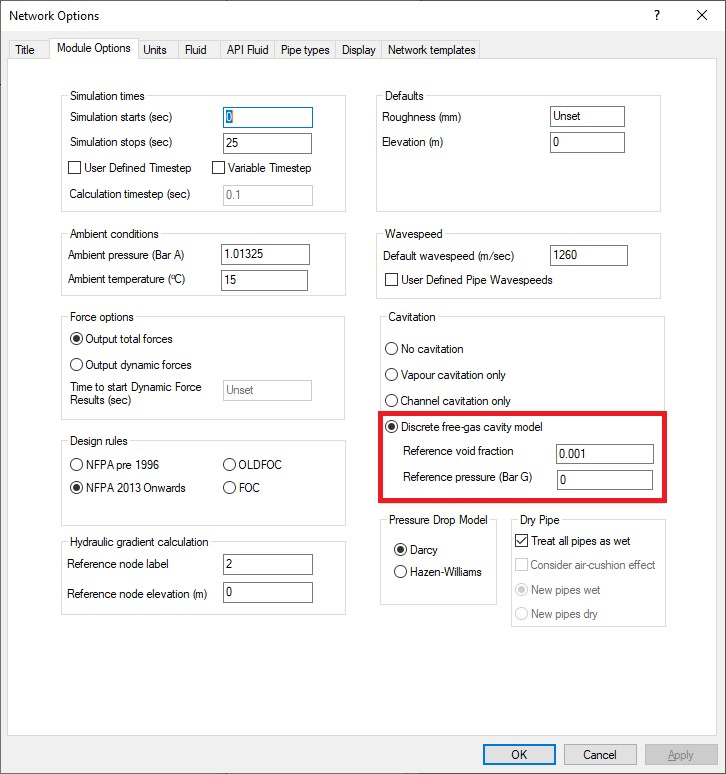Introduction to Discrete Gas Cavity Model (DGCM)

PIPENET Transient Module has developed two cavitation models (vapour cavitation and channel cavitation) for transient phenomena of column separation. Both the models are based on the assumption of the absence of free gas in liquid systems, i.e. single-component two-phase transient flow. However, liquid usually contains a little amount of free gas in many practical physical systems which may produce great impact on wave propagation (speed and magnitude). Wylie and Streeter, who discuss this in the book “Fluid Transients in Systems”, state that it is generally assumed that the mass of free gas remains constant at the initial value during a transient event as it is difficult to predict the dynamic value accurately.

This model proposed by Wylie and Streeter to consider the effect of a small quantity of free gas in continuous liquid, i.e. discrete gas cavity model (DGCM), is available in PIPENET Transient module.

Activating DGCM in PIPENET Transient module

The discrete free-gas cavity model (DGCM) can be activated in “Module Options” window. If the cavitation option is “Discrete free-gas cavity model”, the reference void fraction and reference pressure are required to calculate gas mass in unit volume liquid. The reference void fraction is the gas volume void fraction at the reference pressure.

The default reference void fraction is 0.001. The reference void fraction must be in the range of 0.0 < VF < 1.0. Otherwise, an error message will be given.

The default reference pressure is 0 Bar G. The reference pressure must be bigger than the vapour pressure. If the vapour pressure exceeds 0 Bar G, the default reference pressure is set as vapour pressure + 1 Bar. Otherwise, an error message will be given.